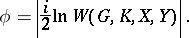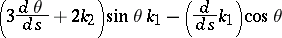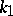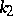# Laguerre formula

(diff) ← Older revision | Latest revision (diff) | Newer revision → (diff)

A formula for calculating the angle between straight lines in Euclidean and pseudo-Euclidean spaces. Letandbe the points at infinity on two straight linesandand letandbe the points of intersection of these lines with the absolute of the space. Then the anglebetween these lines can be expressed in terms of the cross ratio:For two-dimensional pseudo-Euclidean space,andare the direction vectors of the isotropic lines passing through the point of intersection of the linesand.

The formula was introduced by E. Laguerre .

A formula according to which, for all curves on a given surface that touch at some point, the quantityis invariant, whereandare the curvature and the torsion of the curve,is the angle between the principal normal of the curve and the normal to the surface, andis the natural parameter on the curve. The formula was obtained by E. Laguerre (1870, see ).

How to Cite This Entry:
Laguerre formula. Encyclopedia of Mathematics. URL: http://encyclopediaofmath.org/index.php?title=Laguerre_formula&oldid=11758
This article was adapted from an original article by D.D. Sokolov (originator), which appeared in Encyclopedia of Mathematics - ISBN 1402006098. See original article